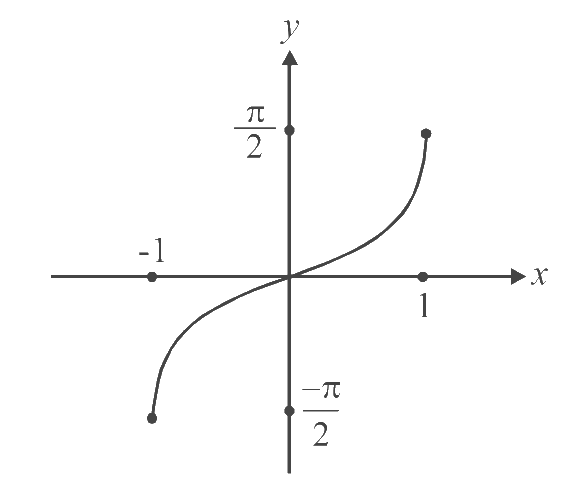# Inverses of Some Standard Functions

Go back to  'Functions'

Lets try to define an inverse for some of the functions that we have seen up till now.

(a)

$$f(x) = x\,\,\,\,\,\,\mathbb{R} \to \mathbb{R}$$

We see that this function is one-one and onto. The inverse exists.

$${f^{ - 1}}(x) = x\,\,\,\,\,\,\mathbb{R} \to \mathbb{R}.$$

(b)

$$f(x) = {x^2}\,\,\,\,\,\,\mathbb{R} \to \mathbb{R}$$

This function is many-one and into. (why?). To define the inverse, we first need to make $$f$$ one-one and onto.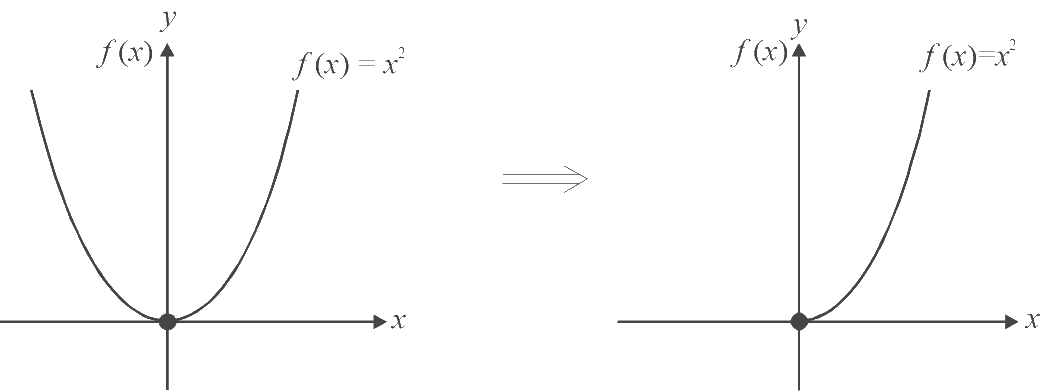We see that to make the function one-one, we can select the domain as only [$$0,\;\infty$$) (instead of $$\mathbb{R}$$).

In this domain, the function is one-one, as is clear from the second diagram above.

Also, the range is [$$0,\;\infty$$). Therefore, redefine this function:

$f(x) = {x^2}\mathop {[0,\infty )}\limits_A {\mkern 1mu} {\mkern 1mu} {\mkern 1mu} \to {\mkern 1mu} {\mkern 1mu} {\mkern 1mu} \mathop {[0,\infty )}\limits_B {\rm{ }}$

This function is now one-one and onto, and hence invertible

${f^{ - 1}}(x){\rm{ }} = {\rm{}}\sqrt x {\rm{ }}\mathop {[0,\infty )}\limits_B {\mkern 1mu} {\mkern 1mu} {\mkern 1mu} \to {\mkern 1mu} {\mkern 1mu} {\mkern 1mu} \mathop {[0,\infty )}\limits_A$

Additionally, note that if we draw the graph of $$\sqrt x$$ and $$x^2$$ on the same axis, they are mirror images of each other in the line $$x=y.$$

(As we saw in the case of $$\,f(x) = \log x$$  and $${f^{ - 1}}(x) = {2^x}).$$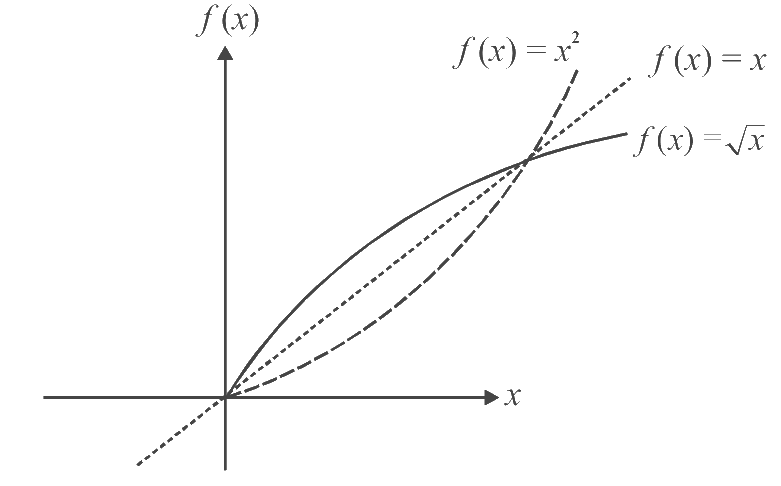A little thought will show why $$f$$ and  $${f^{ - 1}}$$ should be mirror images in the mirror $$y=x.$$

In the equation  $$y = f(x),$$ $$x$$ can be treated as the independent and y the dependent variable.

In the equation $$x = {f^{ - 1}}(y),$$ we can reverse the roles. We can treat $$y\text{-axis}$$ as the independent variable axis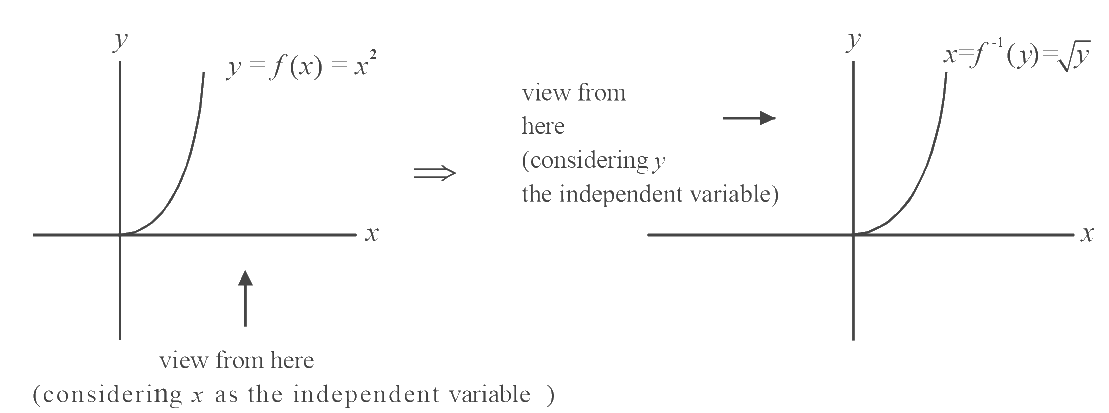By convention, the independent variable is taken on the horizontal axis. Therefore we convert this horizontal view (the second diagram above) into a conventional vertical view. How? By taking the reflection in $$y = x.$$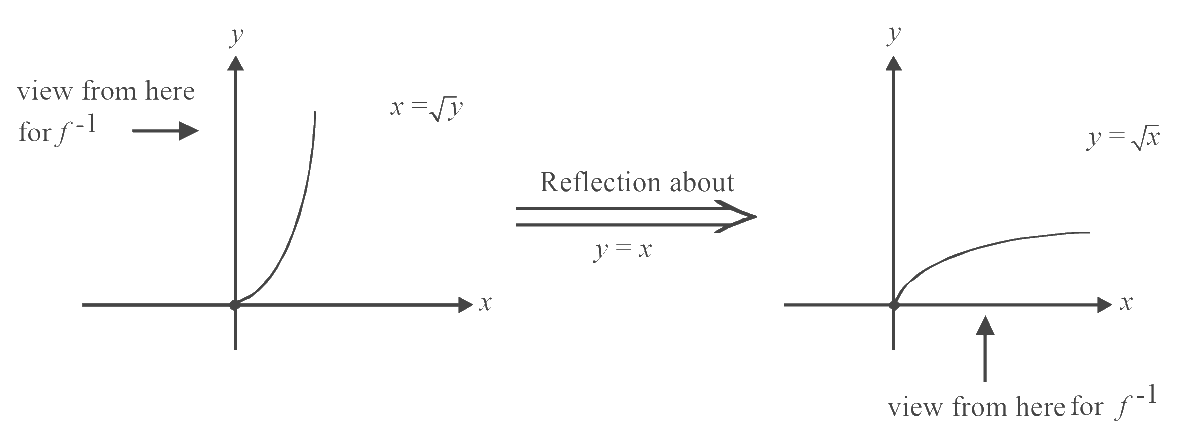(c)   $$f (x)=\text{sin}x\;$$$$\mathbb{R}\;$$$$\rightarrow \;$$$$[–1, 1]$$

This function is onto but not one-one. Lets make it one-one first. Select that interval as the domain, in which $$\text{sin}x$$ remains one-one.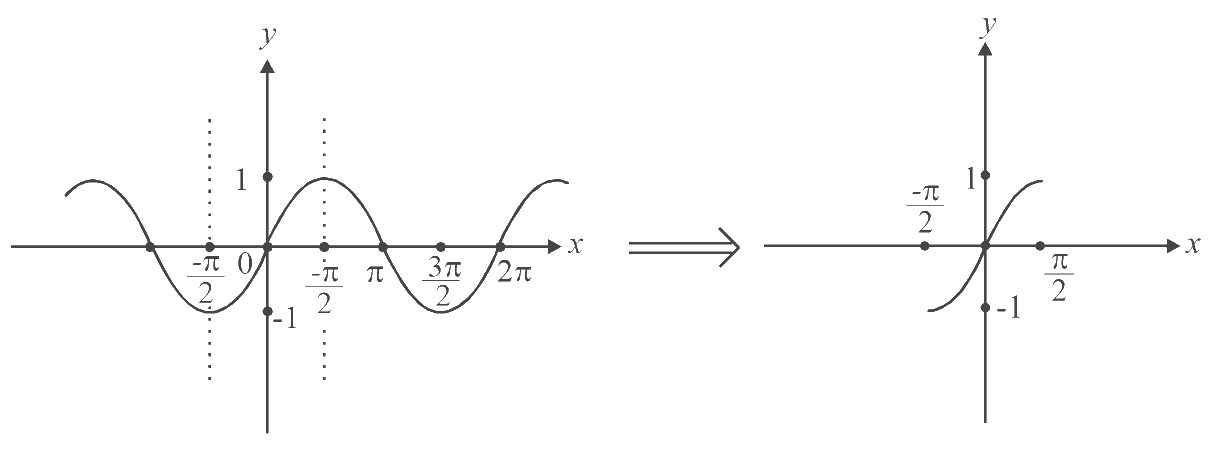In other words, we redefine $$f$$ as \begin{align}f(x) = \sin x\,\,\,\,\,\left[ { - \frac{\pi }{2},\frac{\pi }{2}} \right] \to [ - 1,1]\end{align} (we could have taken any other interval in which $$\text{sin}x$$ remains one-one, to define the inverse. But conventionally, we take the interval closest to the origin; \begin{align}\left[ { - \frac{\pi }{2},\frac{\pi }{2}} \right]\end{align}  in this case, which is centred on the origin). The inverse can now be defined as:

${f^{ - 1}}(x){\rm{}} = {\rm{}}{\sin ^{ - 1}}x\quad [ - 1,1] \to {\rm{}}\left[ { - \frac{\pi }{2},\frac{\pi }{2}} \right]$